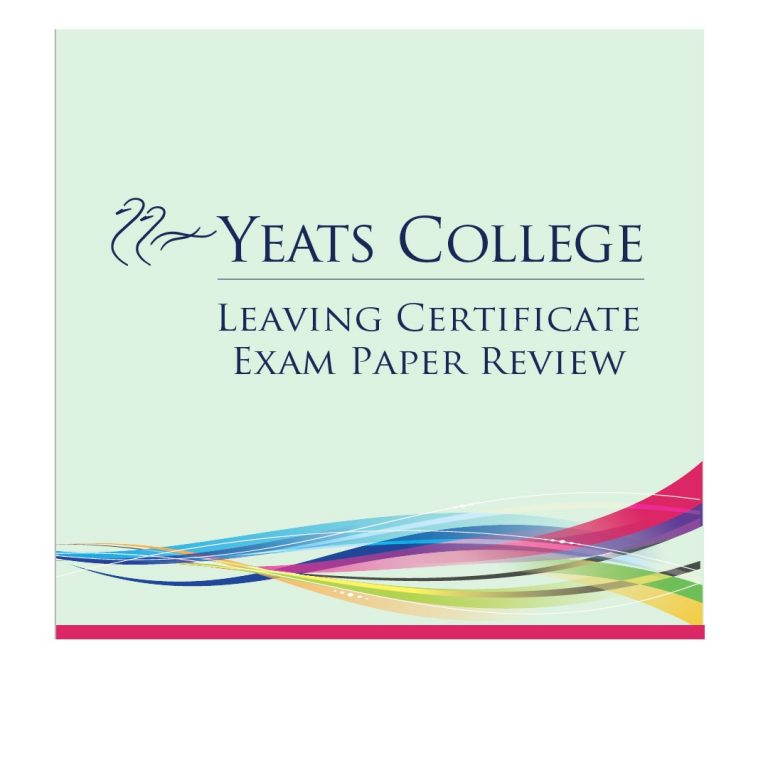# Leaving Certificate Higher Level Maths Papers 1 & 2 Review

## by Aisling Kenny

Friday afternoon’s Maths paper 1 was a very fair exam. Some questions appeared tricky at first, but students generally found that they came out quite nicely once they got started. Students benefited from a lot of choice in this year’s adapted paper. There was certainly an emphasis on calculus throughout the paper.

Section A consisted of six questions and students were required to answer four. The questions included complex numbers, algebra, sequences and series and calculus. The complex numbers question was quite unusual and required an understanding of the geometry of complex numbers.

Students were required to answer two of the four options in Section B. Question 7 was calculus based with some interesting questions in the final part. Question 8 involved a trigonometric function. Question 9 was a very straightforward sequences and series question. Question 10 was a very accessible question involving a log function and calculus.

Monday morning’s paper 2 was not as straightforward, but students were still very happy with the paper. Section A contained questions on probability, the line, the circle, trigonometry and geometry. These included a trigonometric proof and the construction of the circumcentre of a triangle, both of which students were very happy to see. Inferential statistics also appeared in Section A. Section B began with a very straightforward area and volume question, followed by a probability and statistics questions which included a component on correlation. Question 9 required solving trigonometric triangles. This question included parts on speed, distance and time. Question 10 involved probability including z-scores and Bernoulli trials.

Overall, students were very happy with this year’s Maths exam. Some were disappointed and surprised by the absence of financial maths. However, those who had worked consistently were rewarded with a challenging but comprehensive paper.

## Wait!